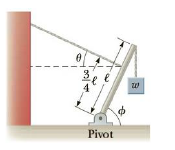Chapter 8, Problem 34P

Chapter
Section
Textbook Problem

A 1200-N uniform boom at Φ = 65° to the horizontal is supported by a cable at an angle θ = 25.0° to the horizontal as shown in Figure P8.34. The boom is pivoted at the bottom, and an object of weight w = 2 000 N hangs from its top. Find (a) the tension in the support cable andFigure P8.34

(a)

To determine
The tension in the cable.

Explanation

Given info: The weight of the uniform boom is 1200N , the weight of the object is 2000N , and the angle of the boom is 65° .

Explanation: The sum of torques on the boom that pivoted at the bottom is τ=0=(l/2)WBcosϕ+(3l/4)TlWcosϕ and this expression is rearranged for the force T .

The formula for the force of tension is,

T=(4/3)Wcosϕ(2/3)WBcosϕ

• W is weight of the object.
• WB is weight of the boom.
• ϕ is the angle of the boom from horizontal

(b)

To determine
The components of the reaction force exerted by the pivot on the boom.

Still sussing out bartleby?

Check out a sample textbook solution.

See a sample solution

The Solution to Your Study Problems

Bartleby provides explanations to thousands of textbook problems written by our experts, many with advanced degrees!

Get Started